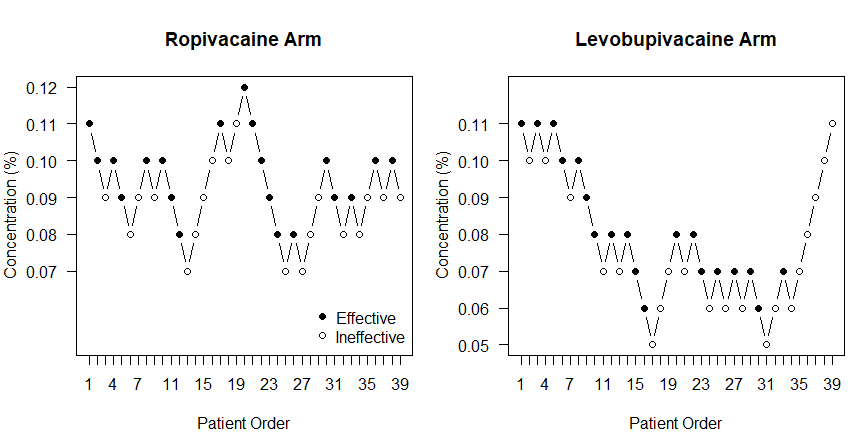# Vignette for ‘cir’ Package

## Background

We demonstrate the cir package with data from the anesthesiology experiment of Benhamou et al.1. This experiment used the Up-and-Down (UD) dose-finding design, and was re-analyzed a few years later by Pace and Stylianou.2 Here we re-analyze them again using state-of-the-art Centered Isotonic Regression (CIR),3 the core estimator found in our package.

The study randomized women in labor into two groups, receiving as analgesic either ropivacaine or levobupivacaine, to estimate each agent’s median effective dose (ED$$_{50}$$) for this condition, and test whether they are different. The original study used a “traditional” dose-averaging estimation method, concluding that even though levobupivacaine seemed 19% more potent, the difference between ED$$_{50}$$’s, and hence the difference in potency, was not significant. To derive inference about this difference, they apparently used the standard errors of the dose averages in a $$t$$-test like manner.

Pace and Stylianou re-analyzed the data using isotonic regression (IR) and 83% bootstrap confidence intervals (CIs); under certain assumptions, examining whether these CIs overlap is equivalent to rejecting the Null hypothesis. 4 They found a larger and statistically significant difference between the two ED$$_{50}$$’s. Per their estimates, levobupivacaine was 37% more potent.

Here we revisit this experiment yet again.

## Experimental Trajectories

Since UD datasets are rather compact, adding the data takes no more than 2-3 lines of code. But you can also use .csv file input if preferred, with two columns headed “x” and “y”. We do not have the original data table, nor do the original authors have access to it anymore; but we can read the sequence of administered doses off of Benhamou et al.’s Figure 1.

The figure sizes have been customised so that you can easily put two images side-by-side.

# For brevity, we initially use integers to denote the doses.
# We make use of R’s shorthand for consecutive sequences,
# e.g., 1:3 is really 1,2,3
xropi = c(11:9,10:8,9,10,9,10:7,8:11,10:12,11:7,8,7:10,9,8,9,8:10,9,10,9,10)
xlevo = c(11,10,11,10,11:9,10:7,8,7,8:5,6:8,7,8:6,7,6,7,6,7:5,6,7,6:12)

The study design being “classical” or median-finding UD, the responses ($$y$$) can be read off directly from the doses ($$x$$): a positive increment indicates no effectiveness ($$y=0$$), and vice versa. Symbolically, $y = \left( 1-diff(x) \right) / 2.$ We use this and the DRtrace() constructor utility, to create objects that store doses (converted to their physical units) and responses; as we call them here, the experimental “trace” or trajectory.

library(cir)
bhamou03ropi = DRtrace(x=xropi[-40]/100, y=(1-diff(xropi))/2)
bhamou03levo = DRtrace(x=xlevo[-40]/100, y=(1-diff(xlevo))/2)

In the construction above, we gave up the 40th and last observation in each arm, because we only know its dose but not the response.

DRtrace objects have a native plotting method:

par(mfrow=c(1,2), las=1, mar=c(4,4,4,1)) # image format parameters
doserange = c(5,12)/100

plot(bhamou03ropi, ylim=doserange, ylab="Concentration (%)", main='Ropivacaine Arm')
legend('bottomright',legend=c('Effective','Ineffective'),pch=c(19,1),bty='n')
plot(bhamou03levo, ylim=doserange, ylab="Concentration (%)", main='Levobupivacaine Arm')## ED$$_{50}$$ Estimates

To derive IR and CIR estimates, we take the DRtrace trajectory objects and generate doseResponse dose-rate-count summaries.

bhamou03ropiRates = doseResponse(bhamou03ropi)
bhamou03levoRates = doseResponse(bhamou03levo)
knitr::kable(bhamou03ropiRates, row.names=FALSE,align='ccr',digits=c(2,4,0))
x y weight
0.07 0.0000 3
0.08 0.3750 8
0.09 0.3846 13
0.10 0.8000 10
0.11 0.7500 4
0.12 1.0000 1
knitr::kable(bhamou03levoRates, row.names=FALSE,align='ccr',digits=c(2,4,0))
x y weight
0.05 0.0000 2
0.06 0.2500 8
0.07 0.5455 11
0.08 0.8333 6
0.09 0.3333 3
0.10 0.4000 5
0.11 0.7500 4

From here, ED$$_{50}$$ estimation is one step away:

ropiTargetCIR=quickInverse(bhamou03ropiRates, target=0.5, adaptiveShrink=TRUE)
ropiTargetCIR
#   target      point lower90conf upper90conf
# 1    0.5 0.09383622  0.08090006   0.1060014
# 1    0.5 0.06842105  0.05395897  0.08389953